# EM.Ferma Tutorial Lesson 3: Modeling Objects Immersed In A Uniform Electric Field

 Tutorial Project: Modeling Objects Immersed In Uniform Electric Fields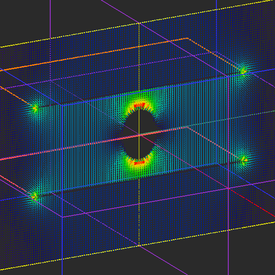Objective: In this project, you will simulate the perturbation of a uniform electric field due to immersion of metallic and dielectric objects. Concepts/Features: PEC Object Dielectric Object Electric Conductivity Parallel Plate Capacitor Uniform Field Distribution Field Sensor Observable Intensity Plot Vector Plot Minimum Version Required: All versions

## What You Will Learn

Previously, you observed that the electric field in the region between the two parallel plates of a capacitor is fairly uniform. In this tutorial you will use parallel metal plates held at different potentials to generate a uniform electric field. Then you will simulate the interaction of this uniform field with immersed objects of different material compositions.

## Getting Started

Open the EM.Cube application and switch to EM.Ferma. Start a new project with the following parameters:

 Name EMFerma_Lesson3 Millimeters N/A (keep default) N/A (keep default) N/A (keep default)

## Creating a Uniform Electric Field

Create three Fixed-potential PEC groups in the navigation tree as follows:

Material Group Material Type Color Fixed Potential
PEC_1 PEC Rosy red (default) -10V
PEC_2 PEC Orange 10V
PEC_3 PEC Pink 0V

Draw the following two rectangle strip objects:

Part Object Type Material Type Dimensions Coordinates Rotation Angles
Rect_Strip_1 Rectangle Strip PEC_1 50mm × 50mm (0, 0, -10mm) (0°, 0°, 0°)
Rect_Strip_2 Rectangle Strip PEC_2 50mm × 50mm (0, 0, 10mm) (0°, 0°, 0°)

Open the domain settings dialog and reduce the domain offset to 15mm in all the six directions. Then open the mesh settings dialog and change the fixed mesh cell size to Δx = Δy = Δz = 0.5mm. Define three field sensor observables according to the table below:

Field Sensor Direction Coordinates Plot Type Max. Size Cone Length Ratio Cone Radius Ratio
Sensor_1 Z (0, 0, 0) Intensity - - -
Sensor_2 X (0, 0, 0) Vector 2 0.75 0.25
Sensor_3 Y (0, 0, 0) Intensity - - -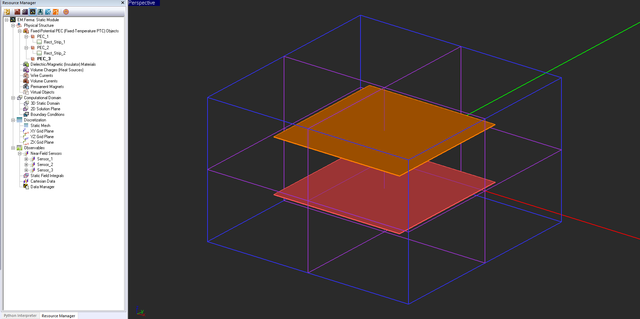The geometry of the two parallel metal plates with different potentials.

Run an electrostatic analysis of your parallel plate structure and visualize the field distributions on the three sensor planes. You can see a uniform electric field of strength |E| = (20V)/(20mm) = 1000V/m at the center plane.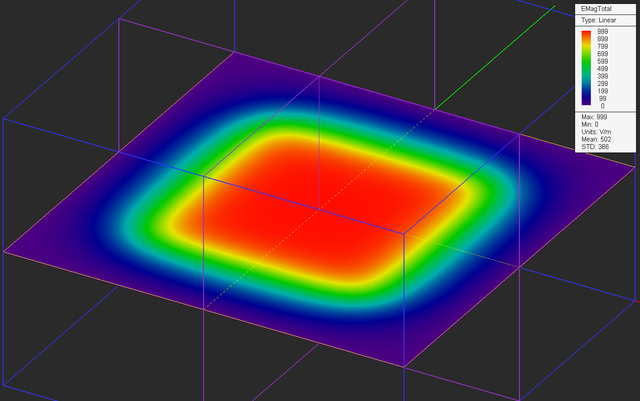The electric field distribution in the Sensor_1 (XY) plane.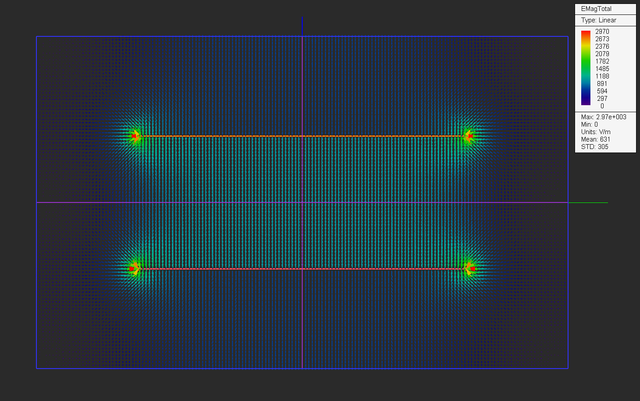The electric field distribution in the Sensor_2 (YZ) plane.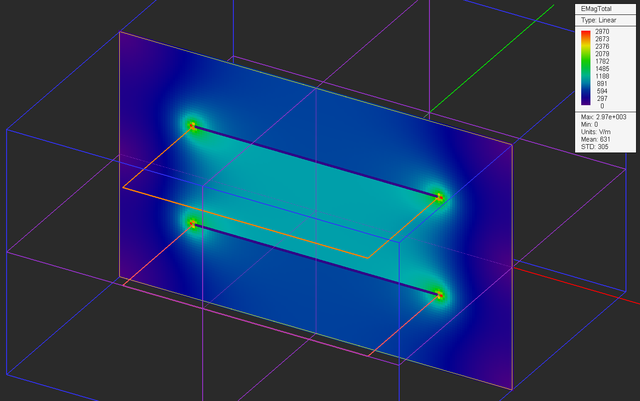The electric field distribution in the Sensor_3 (ZX) plane.

The figures below are the plots of the data files "Sensor_3_X_ETotal.DAT" and "Sensor_3_Z_ETotal.DAT". These show the variation of the electric field along the X and Z axes, respectively.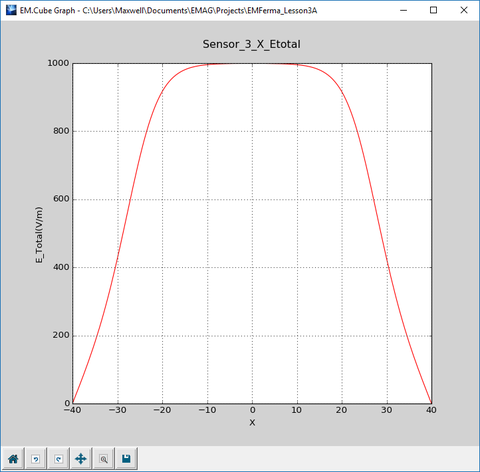The plot of variation of the electric field along the X-axis.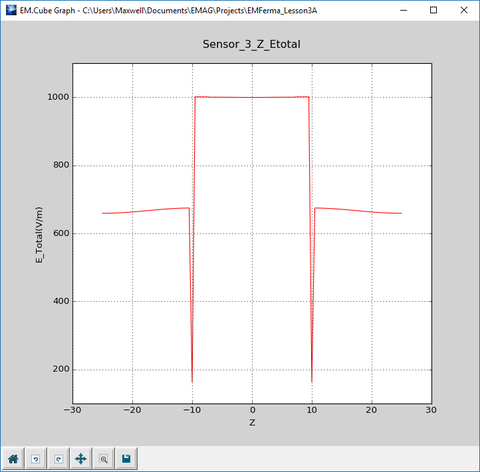The plot of variation of the electric field along the Z-axis.

## Immersing a Metallic Ellipsoid in the Uniform Field

With the PEC_3 group active in the navigation tree, draw a small ellipsoid according to the table below:

Part Name Object Type Material Type Dimensions Coordinates Rotation Angles
Immerse Ellipsoid PEC_3 rx = 4mm, ry = 4mm, rz = 6mm (0, 0, 0) (0°, 0°, 0°)

To draw a ellipsoid, click the Ellipsoid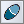button from the Object Toolbar or select the menu item Object → Solid → Ellipsoid .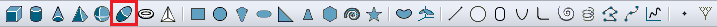Selecting the Ellipsoid tool in the object toolbar.

After drawing the PEC ellipsoid and placing it at the center of the gap region between the two metal plates, your physical structure should look like this: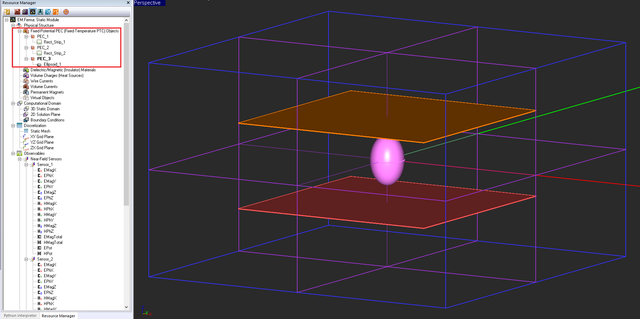The geometry of the two parallel metal plates and an immersed metallic ellipsoid.

Run an electrostatic analysis of your new structure and visualize the field distributions on the three sensor planes.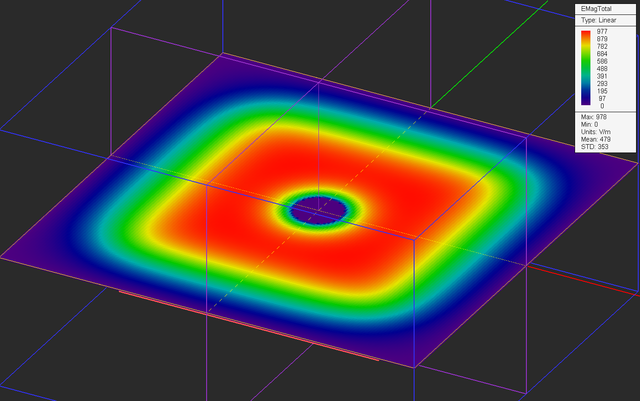The electric field distribution in the Sensor_1 (XY) plane in the presence of the immersed PEC ellipsoid.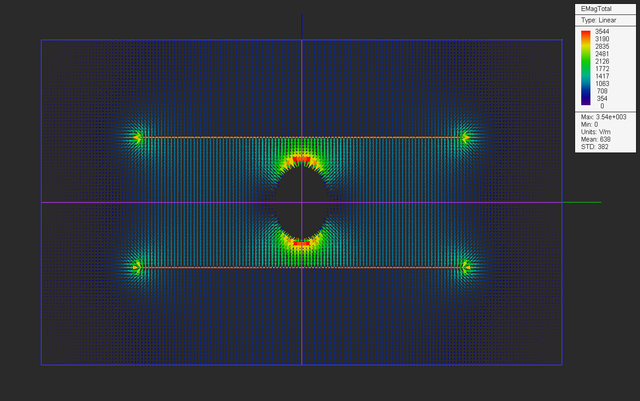The electric field distribution in the Sensor_2 (YZ) plane in the presence of the immersed PEC ellipsoid.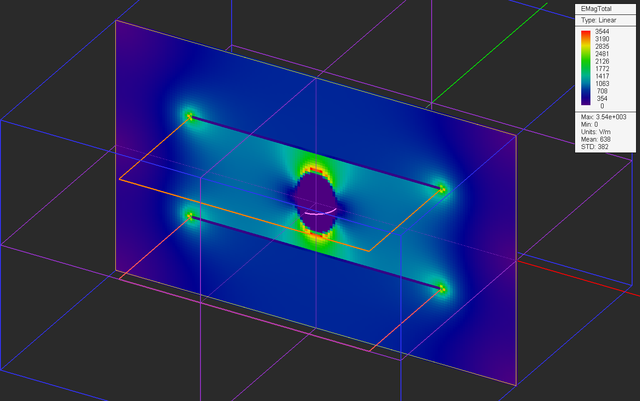The electric field distribution in the Sensor_3 (ZX) plane in the presence of the immersed PEC ellipsoid.

The figures below are the plots of the data files "Sensor_3_X_ETotal.DAT" and "Sensor_3_Z_ETotal.DAT". These show the variation of the electric field along the X and Z axes, respectively.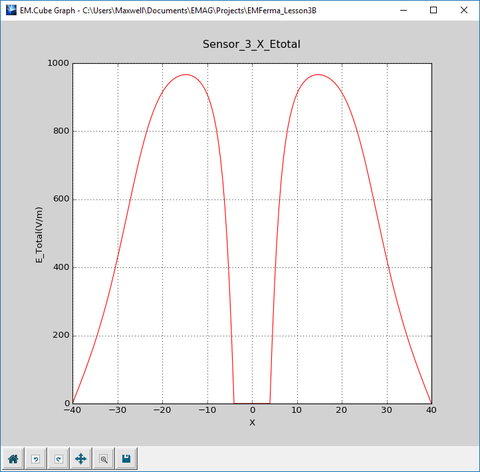The plot of variation of the electric field along the X-axis in the presence of the immersed PEC ellipsoid.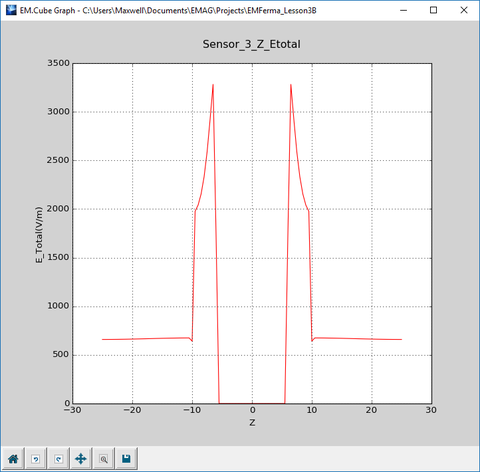The plot of variation of the electric field along the Z-axis in the presence of the immersed PEC ellipsoid.

## Immersing a Lossy Dielectric Ellipsoid in the Uniform Field

Define a new dielectric material group called Dielectric_1 according to the table below:

Material Group Material Type εr μr σ σm
Dielectric_1 Dielectric 4.0 1.0 100.0 0.0

Instead of deleting the PEC ellipsoid and drawing a new dielectric ellipsoid, you can simply move the object "Immerse" from the group "PEC_3" to the group "Dielectric_1". Follow the same procedure as you did in Tutorial Lesson 1 using the Move To items in the contextual menu of the spheroid object.

After drawing the PEC ellipsoid and placing it at the center of the gap region between the two metal plates, your physical structure should look like this: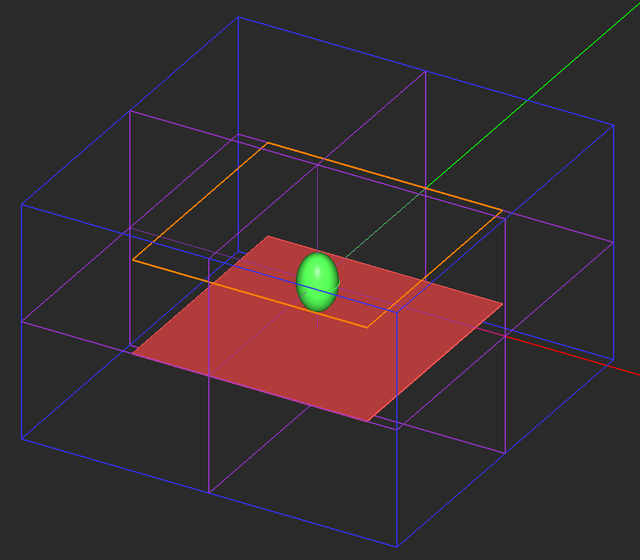The geometry of the two parallel metal plates and an immersed lossy dielectric ellipsoid. The top metal plate is in a freeze state.

Run an electrostatic analysis of your new structure and visualize the field distributions on the three sensor planes.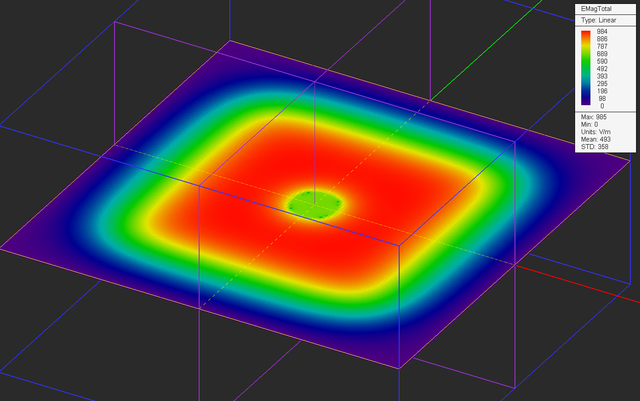The electric field distribution in the Sensor_1 (XY) plane in the presence of the immersed lossy dielectric ellipsoid.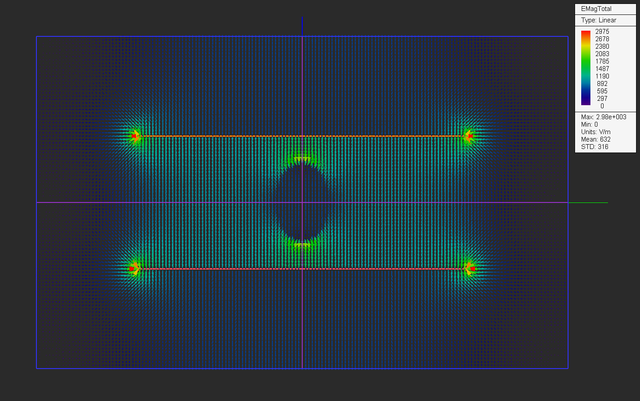The electric field distribution in the Sensor_2 (YZ) plane in the presence of the immersed lossy dielectric ellipsoid.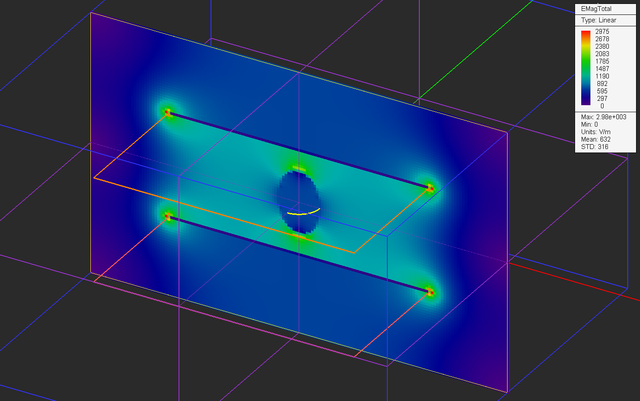The electric field distribution in the Sensor_3 (ZX) plane in the presence of the immersed lossy dielectric ellipsoid.

The figures below are the plots of the data files "Sensor_3_X_ETotal.DAT" and "Sensor_3_Z_ETotal.DAT". These show the variation of the electric field along the X and Z axes, respectively.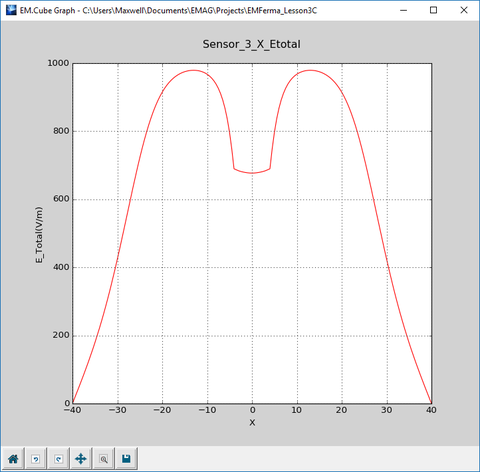The plot of variation of the electric field along the X-axis in the presence of the immersed lossy dielectric ellipsoid.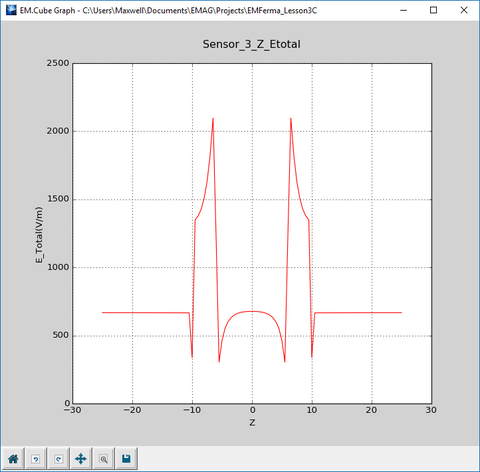The plot of variation of the electric field along the Z-axis in the presence of the immersed lossy dielectric ellipsoid.

## Analyzing the Field Discontinuities

Let's take a look at the graphs of variation of the electric field along the X-axis in the three different cases you investigated above. In the first case without any immersed object, you see a uniform electric field of 1000V/m across the region between the two metal plates, which rolls off quickly in the outside free space region. In the second case with an immersed PEC ellipsoid, there is an actual null field at the center inside the ellipsoid. In the third case with an immersed dielectric ellipsoid, there is a non-zero field of a reduced amplitude.

Now look at the variation of the electric field along the Z-axis in the third case with the dielectric spheroid. There is a sharp discontinuity at Z = ±6mm, which represent the interfaces between the air and your dielectric material. This is expected as a result of the governing boundary conditions between two different dielectric materials. The boundary conditions require that:

$D_{n1} = D_{n2}$

or

$\epsilon_{r1} E_{n1} = \epsilon_{r2} E_{n2}$

In the case of your dielectric material, this means that

$\frac{E_{z1}}{E_{z2}} = 4$

In the data manager, view the contents of the data file "Sensor_3_Z_ETotal.DAT" in the spreadsheet as shown below.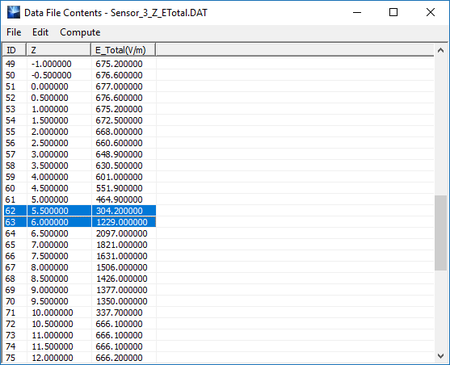The contents of the data file "Sensor_3_Z_ETotal.DAT" shown in the data manager's spreadsheet.

Read the field values at the points below and above the dielectric-air interface. You can see that the computed ratio agrees well with the analytical result.

$\frac{E_{z1}}{E_{z2}} = \frac{|E(6mm)|}{|E(5.5mm)|} = \frac{1229.00}{304.20} = 4.04$